# JP5704249B2 - Observation information processing apparatus, observation information processing program, and observation information processing method - Google Patents

Observation information processing apparatus, observation information processing program, and observation information processing method Download PDF

## Info

Publication number
JP5704249B2
JP5704249B2 JP2013535952A JP2013535952A JP5704249B2 JP 5704249 B2 JP5704249 B2 JP 5704249B2 JP 2013535952 A JP2013535952 A JP 2013535952A JP 2013535952 A JP2013535952 A JP 2013535952A JP 5704249 B2 JP5704249 B2 JP 5704249B2
Authority
JP
Japan
Prior art keywords
mesh
set
observation information
information processing
Prior art date
Legal status (The legal status is an assumption and is not a legal conclusion. Google has not performed a legal analysis and makes no representation as to the accuracy of the status listed.)
Active
Application number
JP2013535952A
Other languages
Japanese (ja)
Other versions
JPWO2013046747A1 (en
Inventor

Original Assignee

Priority date (The priority date is an assumption and is not a legal conclusion. Google has not performed a legal analysis and makes no representation as to the accuracy of the date listed.)
Filing date
Publication date
Priority to JPPCT/JP2011/072572 priority Critical
Priority to PCT/JP2011/072572 priority patent/WO2013046435A1/en
Application filed by 富士通株式会社 filed Critical 富士通株式会社
Priority to PCT/JP2012/054283 priority patent/WO2013046747A1/en
Priority to JP2013535952A priority patent/JP5704249B2/en
Publication of JPWO2013046747A1 publication Critical patent/JPWO2013046747A1/en
Application granted granted Critical
Publication of JP5704249B2 publication Critical patent/JP5704249B2/en
Application status is Active legal-status Critical
Anticipated expiration legal-status Critical

## Images

••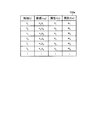•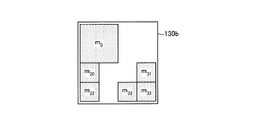•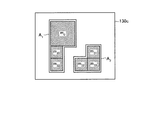•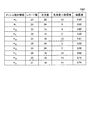•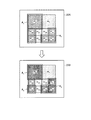•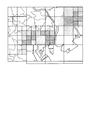•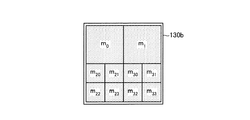•••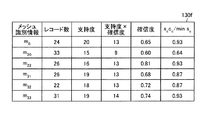•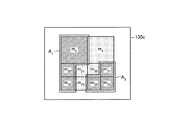••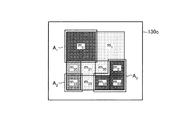•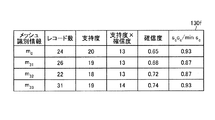•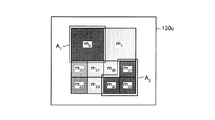•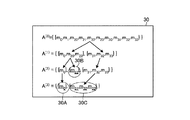•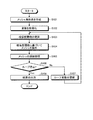••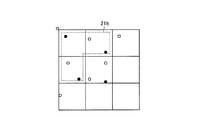•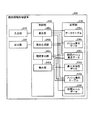•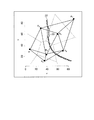•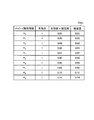•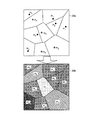•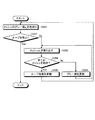•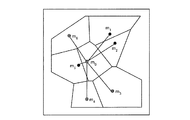•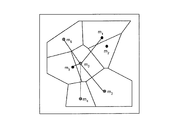•••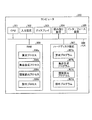•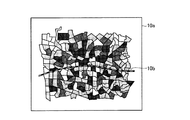•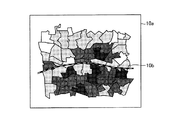•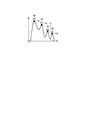## Description

The present invention relates to an observation information processing apparatus and the like.

Conventionally, data is aggregated by dividing the area into meshes. 31 and 32 are diagrams showing an example of conventional data aggregation. 31 divides a certain area 10a by a mesh for each town chome, and FIG. 32 divides the area 10a by a mesh obtained by combining the four adjacent meshes of FIG. 31 and 32, the darker the mesh, the greater the population.

When FIG. 31 and FIG. 32 are compared, even in the same region 10a, a change occurs in the obtained result depending on how the mesh is divided. For example, in FIG. 31, it seems that the north of the railway 10b has a large population. On the other hand, in FIG. 32, it seems that the south of the railway 10b has a large population. Such a change in the result obtained by dividing the mesh is called a variable unit district problem.

In contrast to the above-described conventional technique, there is a conventional technique for setting a correlation rule and detecting a region that maximizes the certainty of the correlation rule. In this prior art, since the mesh division method is not determined in advance, the above variable unit area problem does not occur.

Sugiura Yoshio, "Geographical space analysis", Asakura Shoten, 2003 T. Fukuda et.al, Data mining using two-dimensional optimized association rules, SIGMOD'96 R. Rastogi, Mining optimized association rules with categorical and numeric attributes, IEEE Trans. On Knowledge and Data Engineering, Vol. 14, Issue 1., 2002

However, the above-described conventional technique has a problem that it is not possible to detect a region with the maximum certainty regarding the association rule.

FIG. 33 is a diagram for explaining the concept of local maximum. For example, the horizontal axis corresponds to the position, and the vertical axis corresponds to the certainty factor. Reference numerals 1a to 1d in FIG. 33 denote maximum points. The maximum point 1a is a point having a maximum value in the vicinity 2a. At the maximum point 1a, the value changes from increasing to decreasing. Similarly, the local maximum points 1b to 1d are points having the maximum values in the neighborhoods 2b to 2d, respectively.

In the prior art, a region where the certainty factor is maximized has been detected. The problem of the prior art will be described with reference to FIG. 33. Since the point with the maximum certainty is the local maximum point 1a, the prior art has detected a region corresponding to the local maximum point 1a. However, the conventional technique cannot detect the region corresponding to the remaining maximum points 1b to 1d.

For example, in the case where data is collected for areas where there are many long-distance riders among taxi riders, not only are areas that have the greatest possibility of long-distance rides, but also areas that are maximal. It is convenient if you can show them at the same time. For example, in the maximal area, the possibility of picking up long-distance passengers is not the highest, but there is a possibility of picking up long-distance passengers near the point where the taxi is currently traveling. Because there is. On the other hand, in the prior art, it is possible to detect only a region where the possibility of long-distance riding is maximized.

The present invention has been made in view of the above, and an object of the present invention is to provide an observation information processing apparatus, an observation information processing program, and an observation information processing method capable of detecting a region with a maximum certainty of association rules. To do.

The disclosed observation information processing apparatus includes a calculation unit, a set generation unit, a threshold value calculation unit, and an exclusion unit. The calculation unit calculates, for each mesh, the number of records for which the condition part of the correlation rule is true as the support level, and the ratio of the records in which the result part is also true among the records for which the condition part of the correlation rule is true is used as the certainty factor. Calculate for each mesh. The set generation unit generates an adjacent mesh set by grouping adjacent meshes into a subset of meshes. The threshold calculation unit calculates the certainty factor for each adjacent mesh set based on the support level and the certainty factor of each mesh included in the adjacent mesh set, and sets a minimum certainty factor among the certainty factors as a new certainty factor threshold value. Set as. The exclusion unit detects and excludes meshes to be excluded from the meshes included in the adjacent mesh set based on the certainty factor and the support degree of each mesh included in the adjacent mesh set, and the certainty threshold value.

According to the disclosed observation information processing apparatus, it is possible to detect an area having a maximum certainty about the association rule.

FIG. 1 is a functional block diagram of the configuration of the observation information processing apparatus according to the first embodiment. FIG. 2 is a diagram illustrating an example of the data structure of the data table according to the first embodiment. FIG. 3 is a diagram illustrating an example of the data structure of the mesh set data. FIG. 4 is a diagram illustrating an example of a data structure of adjacent mesh set data. FIG. 5 is a diagram illustrating an example of a data structure of the mesh aggregation table. FIG. 6 is a diagram for explaining the main points of the control unit. FIG. 7 is a diagram illustrating an example of an output result of the output unit. FIG. 8 is a diagram (1) for explaining the processing of the control unit according to the first embodiment. FIG. 9 is a diagram (2) for explaining the process of the control unit according to the first embodiment. FIG. 10 is a diagram (3) illustrating the processing of the control unit according to the first embodiment. FIG. 11 is a diagram (4) illustrating the processing of the control unit according to the first embodiment. FIG. 12 is a diagram (5) for explaining the process of the control unit of the first embodiment. FIG. 13 is a diagram (6) illustrating the processing of the control unit according to the first embodiment. FIG. 14 is a diagram (7) illustrating the processing of the control unit according to the first embodiment. FIG. 15 is a diagram (8) illustrating the processing of the control unit according to the first embodiment. FIG. 16 is a diagram (9) illustrating the processing of the control unit according to the first embodiment. FIG. 17 is a diagram illustrating an example of a tree structure of an adjacent mesh set. FIG. 18 is a flowchart of a process procedure of the observation information processing apparatus according to the first embodiment. FIG. 19 is a diagram (1) illustrating an example of mesh analysis. FIG. 20 is a diagram (2) illustrating an example of mesh analysis. FIG. 21 is a diagram illustrating the configuration of the observation information processing apparatus according to the second embodiment. FIG. 22 is a diagram illustrating an example of Voronoi division and Delaunay graph. FIG. 23 is a diagram illustrating an example of the data structure of the mesh aggregation table according to the second embodiment. FIG. 24 is a diagram for explaining the concept of graying. FIG. 25 is a flowchart illustrating a graying process. FIG. 26 is a diagram (1) illustrating an example of a mesh to be processed by the calculation unit. FIG. 27 is a diagram (2) illustrating an example of a mesh to be processed by the calculation unit. FIG. 28 is a diagram illustrating an operation example in which the optimum region is repeatedly extracted. FIG. 29 is a diagram illustrating an example of the inclusion relationship. FIG. 30 is a diagram illustrating an example of a computer that executes an observation information processing program. FIG. 31 is a diagram (1) illustrating an example of conventional data aggregation. FIG. 32 is a diagram (2) illustrating an example of conventional data aggregation. FIG. 33 is a diagram for explaining the concept of local maximum.

Embodiments of an observation information processing apparatus, an observation information processing program, and an observation information processing method according to the present invention will be described below in detail with reference to the drawings. Note that the present invention is not limited to the embodiments.

An example of the configuration of the observation information processing apparatus according to the first embodiment will be described. In the first embodiment, as an example, a case will be described in which an area where a long taxi ride is frequent is extracted. FIG. 1 is a functional block diagram of the configuration of the observation information processing apparatus according to the first embodiment. As illustrated in FIG. 1, the observation information processing apparatus 100 includes an input unit 110, an output unit 120, a storage unit 130, and a control unit 140.

The input unit 110 is an input device that inputs various data. For example, the user uses the input unit 110 to input the data table 130a, mesh set data 130b, correlation rule data 130d, minimum support data 130e, and the like. The input unit 110 corresponds to a keyboard, a mouse, a touch panel, or the like. The output unit 120 is an output device that outputs the processing result of the control unit 140. For example, the output unit 120 corresponds to a display, a touch panel, or the like.

The storage unit 130 stores a data table 130a, mesh set data 130b, and adjacent mesh set data 130c. In addition, the storage unit 130 stores correlation rule data 130d, minimum support level data 130e, and a mesh aggregation table 130f. The storage unit 130 corresponds to, for example, a semiconductor memory device such as a random access memory (RAM), a read only memory (ROM), and a flash memory, or a storage device such as a hard disk or an optical disk.

The data table 130a includes a plurality of records each having a set of time (t), coordinates (x, y), attribute 1 (v), and attribute 2 (w). For example, the data table 130a is defined as “D = {(t, x, y, v, w)}”. t is defined as “tεT (time)”. x and y are defined as “(x, y) εR 2 (coordinates)”. v and w are defined as “v, wεZ (integer, real number, item value)”.

FIG. 2 is a diagram illustrating an example of the data structure of the data table according to the first embodiment. As shown in FIG. 2, the data table 130a stores time (t), coordinates (x, y), attribute 1 (v), and attribute 2 (w) in association with each other.

For example, in attribute 1 (v), a value is set according to whether or not a taxi is taken. In the case of boarding, “1” is set in attribute 1 (v). In case of getting off, “0” is set in attribute 1 (v).

In attribute 2 (w), a value is set according to whether or not it is a long-distance ride. In the case of long-distance riding, “1” is set. In the case of a short distance ride, “0” is set.

The mesh set data 130b is mesh set data. The mesh set is defined as “M = {m c | m c ∩m d = Φ if c ≠ d”. That is, the meshes that are elements of the mesh set do not overlap each other. Further, an adjacency relationship is defined for a set of meshes that are elements of the mesh set. That is, that two different meshes m c and m d are adjacent denoted as m c ~m d. For example, it may be a m c ~m d when m c and m d are shared boundaries. It should be noted that the mesh shape is not limited to a rectangle and the sizes may be different as long as they do not overlap each other and the adjacency relationship is defined.

The mesh and the mesh set represent the set of records in the mesh and are treated as equivalent to the record set. If “S = {(t, x, y, v, w) | (x, y) εm c }”, instead of calling the record set S, the record set m c may be called. In addition, ∃m c εMs. t. (X, y) ∈m c become possible simply (x, y) ∈M to write. At this time, if “S = {(t, x, y, v, w) | (x, y) εM}”, the record set M may be called instead of the record set S.

FIG. 3 is a diagram illustrating an example of the data structure of the mesh set data. In the example illustrated in FIG. 3, the mesh set data 130 b includes meshes m 0 , m 20 , m 22 , m 31 , m 32 , and m 33 . Here, for convenience of explanation, a rectangular mesh is shown, but the present invention is not limited to this. Each mesh has an adjacency defined. For example, it is defined that m 0 and m 20 are adjacent to each other, and that m 20 and m 22 are adjacent to each other. Further, it is defined that m 31 and m 33 are adjacent to each other, and that m 32 and m 33 are adjacent to each other.

The association rule data 130d is association rule data. For example, the correlation rule is “C 1 (t, x, y, v, w) → C 2 (t, x, y, v, w)”. In this correlation rule, referred to as C 1 and condition part. The C 2 is referred to as a consequence part. Also, “→” in the association rule is an implication. Condition part C 1 and consequence part C 2 is any logical expression about the arguments. When an argument is given, the association rule is either {T (true: True) or F (false: False)}. Hereinafter appropriate, simply referred to as C 1 condition part C 1, simply referred to as C 2 consequences portion C 2.

In the first embodiment, as an example, the conditional part C 1 is “T” when the attribute 1 (v) is “1”, and “F” when the attribute 1 (v) is “0”. Let it be an expression. The consequent part C 2 is a logical expression that is “T” when the attribute 2 (w) is “1” and “F” when the attribute 2 (w) is “0”.

The minimum support level data 130e is data of the minimum support level (minSup). The minimum support level is a constant preset by the user. The control unit 140, which will be described later, uses the minimum support level in the process of detecting a region where the certainty factor regarding the association rule is maximized. The degree of support will be described later.

The mesh aggregation table 130f is used in the process in which the control unit 140 described later detects a region where the certainty factor regarding the association rule is maximized. FIG. 5 is a diagram illustrating an example of a data structure of the mesh aggregation table. As illustrated in FIG. 5, the mesh aggregation table 130 f associates mesh identification information, the number of records, the support level, the support level × the certainty level, and the certainty level. The mesh identification information is information for uniquely identifying a mesh. The number of records indicates the number of records included in the mesh. The support level and the certainty level will be described later.

Subsequently, terms are defined before the description of the control unit 140.

Define the size of the set. The size of the set is the number of elements belonging to the set S. The size of the set is denoted as | S |.

The size of the subset that satisfies the logical expression will be described. Of the record set S, the size of the subset satisfying the logical expression C is defined as follows.
# (C | S) = | {(t, x, y, v, w) | (t, x, y, v, w) εS∧C (t, x, y, v, w) = T} ｜

Since the mesh and the record set are equivalent, they can be defined as follows.
# (C | m c ) = | {(t, x, y, v, w) | (x, y) ∈m c ∧C (t, x, y, v, w) = T} |
# (C | A j ) = | {(t, x, y, v, w) | (x, y) εA j ∧C (t, x, y, v, w) = T} |

The support level (Support) will be described. Support of, among the record set S, is the number of records that condition part C 1 in association rule is true. The degree of support is defined as follows.
sup (C 1 → C 2 | S) = # (C 1 | S)

When only one association rule is handled, the support level is appropriately expressed as sup (S). Since the mesh and the record set are equivalent, they can be defined as follows.
sup (m c ) = # (C | m c )
sup (A j ) = # (C | A j )

The confidence level (Confidence) will be described. Confidence, among the records condition part C 1 in association rule is true, consequence part C 2 is also a ratio of the record to be true. The confidence is defined as follows.
conf (C 1 → C 2 | S) = # (C 1 ∧C 2 | S) / # (C 1 | S)

When only one association rule is handled, the certainty factor is appropriately expressed as conf (S).

The following relationship holds between the support level and the certainty level.
sup (C 1 → C 2 | S) conf (C 1 → C 2 | S) = # (C 1 ∧C 2 | S)

Since the mesh and the record set are equivalent, they can be defined as follows.
conf (m c ) = # (C 1 ∧C 2 | m c ) / # (C 1 | m c )
conf (A j ) = # (C 1 ∧C 2 | A j ) / # (C 1 | A j )

The maximum region of certainty will be described. When the adjacent mesh set A j satisfies the following property, the certainty level is said to be maximum.
sup (A j )> minSup and conf (A j ) <conf (A j ) for ∀A j ⊃A j

Next, processing of the control unit 140 will be described. The control unit 140 acquires a data table 130a, mesh set data 130b, correlation rule data 130d, and minimum support data 130e from the input unit 110.

The control unit 140 calculates the support level and the certainty factor of each mesh from the data table 130a. The control unit 140 updates the certainty threshold value, and excludes the meshes included in the adjacent mesh set from the meshes included in the adjacent mesh set based on the certainty factor and the support level of each mesh included in the adjacent mesh set. Are detected and excluded.

The main point of the process in which the control unit 140 excludes the mesh based on the certainty factor threshold will be described. FIG. 6 is a diagram for explaining the main points of the control unit. As a premise, as shown in 20A, it is assumed that adjacent mesh sets A 1 and A 2 are detected.

The adjacent mesh set A 1 includes meshes m 0 , m 20 , and m 22 .
A 1 = {m 0 , m 20 , m 22 }

The adjacent mesh set A 2 includes meshes m 31 , m 32 , and m 33 .
A 2 = {m 31 , m 32 , m 33 }

The support level of the mesh m c is s c = sup (m c ), and the certainty level is c c = conf (m c ). The confidence threshold (minConf) is defined as follows. Confidence threshold of confidence and credibility of the adjacent mesh set A 2 adjacent mesh set A 1, the minimum confidence.
minConf = min {conf (A 1 ), conf (A 2 )}

The minimum support value (min c ) is defined as follows. The minimum support level is the minimum support level among s 0 , s 20 , s 22 , s 31 , s 32 , and s 33 .
mins c = min {s 0 , s 20 , s 22 , s 31 , s 32 , s 33 }

Here, even if a mesh that does not satisfy the following conditions exists in the adjacent mesh set, the certainty factor of the adjacent mesh set does not increase.
s c c c / mins c ≧ minConf ··· conditions

The control unit 140 excludes meshes that do not satisfy the condition from meshes included in the adjacent mesh sets A 1 and A 2 . For example, the mesh m 20 adjacent mesh set A 1 is, if the condition is not satisfied, the control unit 140, the mesh m 20, excluded from the adjacent mesh set A 1.

When the control unit 140 excludes the mesh m 20 from the adjacent mesh set A 1 , the result is 20B in FIG. As shown by 20B in FIG. 6, adjacent mesh sets A 1 , A 2 , A 3 are obtained. The control unit 140 detects a region where the certainty factor is maximized by repeatedly executing the processing described with reference to FIG. 6.

Returning to the description of FIG. The control unit 140 includes a calculation unit 140a, a set generation unit 140b, a threshold calculation unit 140c, and an exclusion unit 140d. The control unit 140 corresponds to an integrated device such as an ASIC (Application Specific Integrated Circuit) or an FPGA (Field Programmable Gate Array). The control unit 140 corresponds to an electronic circuit such as a CPU or MPU (Micro Processing Unit).

The calculation unit 140a is a processing unit that counts records for each mesh based on the data table 130a and the mesh set data 130b, and calculates the number of records, the support level, and the certainty level. The calculation unit 140a associates the mesh identification information, the number of records, the support level, the support level × the reliability level, and the reliability level, and stores them in the mesh aggregation table 130f.

The calculation unit 140a compares the coordinates of the data table 130a with the mesh area of the mesh set data 130b, and calculates the number of records included in the mesh. Further, calculation unit 140a, the records contained in the mesh, to calculate the number of records condition part C 1 in association rule is "T" as support of. Further, in the records included in the mesh, the ratio of the records in which the consequent part C 2 is also “T” among the records in which the condition part C 1 of the association rule is “T” is calculated as the certainty factor. In addition, the calculation unit 140a calculates the support level × the certainty factor by multiplying the support factor and the certainty factor.

The set generation unit 140b is a processing unit that generates adjacent mesh set data 130c based on the mesh set 130b and the mesh aggregation table 130f. The set generation unit 140b groups the meshes corresponding to the mesh identification information remaining in the mesh aggregation table 130f based on the adjacency defined by the mesh set data 130b, and generates the adjacent mesh set data 130c.

The threshold calculation unit 140c calculates a certainty factor for each adjacent mesh set based on the adjacent mesh set data 130c and the mesh aggregation table 130f. The threshold value calculation unit 140c outputs the minimum certainty factor among the certainty factors as the certainty factor threshold value to the exclusion unit 140d.

The exclusion unit 140d is a processing unit that detects and excludes meshes to be excluded based on the adjacent mesh set data 130c, the mesh aggregation table 130f, and the minimum support level data 130e.

Processing of the exclusion unit 140d will be described. First, the exclusion unit 140d compares the support levels of the mesh aggregation table with the support levels of the minimum support level data 130e, and specifies the minimum support level (mins c ). The exclusion unit 140d calculates a value “s c c c / mins c ” obtained by dividing the value of “support level × confidence” in the mesh aggregation table 130f by “mins c ”. The excluding unit 140d calculates “s c c c / mins c ” for each mesh identification information in the mesh aggregation table 130f.

The exclusion unit 140d detects mesh identification information in which “s c c c / mins c ” is less than a certainty threshold value as an exclusion target. The excluding unit 140d excludes the record corresponding to the detected mesh identification information from the mesh aggregation table 130f.

Incidentally, the excluding unit 140d performs processing to delete the sup (A i) <a minSup m c ∈A i.

The calculation unit 140a, the set generation unit 140b, the threshold value calculation unit 140c, and the exclusion unit 140d included in the control unit 140 repeatedly execute the above processing until there is no mesh to be excluded.

For example, the exclusion unit 140d superimposes the remaining mesh set remaining in the adjacent mesh set data 130c and the map data after the mesh to be excluded disappears, and outputs it to the output unit 120. FIG. 7 is a diagram illustrating an example of an output result of the output unit. The excluding unit 140d may output a mesh excluding the mesh while gradually changing the color. In this case, the mesh displayed in a dark color corresponds to a region where the certainty factor is maximized. Further, as shown in FIG. 7, the exclusion unit 140d does not always output a mesh, but displays the last excluded mesh in a light color and the last excluded mesh in a dark color. May be. Note that the parent-child relationship of trees is a contour-like relationship on a two-dimensional plane. The control unit 240 may output the parent-child relationship of the tree in the form of contour lines.

Next, the process of the control unit 140 will be described in more detail. 8-16 is a figure for demonstrating the process of the control part of Example 1. FIG. Here, the data table 130a is shown in FIG. 2, and the initial value of the mesh set data 130b is shown in FIG. In addition, it is assumed that the minimum support level (minSup) of the minimum support level data 130e is set to “16”.

The control unit 140 uses the calculation unit 140a to generate the mesh aggregation table 130f based on the data table 130a and the mesh set data 130b. The mesh aggregation table 130f is as shown in FIG.

The control unit 140 initializes the variables M and A as follows. The variable M corresponds to the mesh remaining in the mesh aggregation table 130f. Variable A corresponds to the adjacent mesh set. The numbers attached to the upper right of the variables M and A indicate the number of loops. It becomes 0 in the initial stage.
M (0) = M = {m 0 , m 1 , m 20 , m 21 , m 22 , m 23 , m 30 , m 31 , m 32 , m 33 }
A (0) = {{m 0 , m 1 , m 20 , m 21 , m 22 , m 23 , m 30 , m 31 , m 32 , m 33 }} = {A 1 }

The control unit 140 uses the threshold value calculation unit 140c to update the certainty factor threshold value based on the adjacent mesh set data 130c and the mesh aggregation table 130f. The certainty factor threshold value is obtained as follows and becomes “0.39”.
minConf = min {(13 + 0 + 9 + 0 + 13 + 0 + 0 + 13 + 13 + 14) / (20 + 24 + 15 + 14 + 16 + 24 + 24 + 19 + 18 + 19)}
= 0.39

When the control unit 140 obtains the minimum support level (mins c ) from the support levels of the mesh aggregation table 130f using the exclusion unit 140d, the minimum support level is “14”. The control unit 140 calculates s c c c / mins c for each mesh identification information and registers it in the mesh aggregation table 130f. The mesh aggregation table 130f is as shown in FIG.

The control unit 140 further uses the exclusion unit 140d to detect a record in which the value of s c c c / min c is less than the certainty threshold (0.39) in the mesh aggregation table 130f illustrated in FIG. And exclude. In the example shown in FIG. 10, records corresponding to the mesh identification information m 1 , m 21 , m 23 , and m 30 are excluded, and the mesh aggregation table 130f is as shown in FIG.

Using the set generation unit 140b, the control unit 140 executes mesh connection processing based on the mesh identification information remaining in the mesh aggregation table 130f and the mesh set data 130b, and updates the adjacent mesh set data 130c. . As illustrated in FIG. 12, the control unit 140 performs mesh connection processing, whereby the adjacent mesh sets become A (1) 1 and A (2) 2 . In addition, the control unit 140 updates the variables M and A as follows.
A (1) 1 = {m 0 , m 20 , m 22 }
A (1) 2 = {m 31 , m 32 , m 33 }
A (1) = {A (1) 1 , A (1) 2 }
M (1) = {m 0 , m 20 , m 22 , m 31 , m 32 , m 33 }

Since at least one mesh is excluded by the exclusion unit 140d and the previous variable M (0) is different from the current variable M (1) , the control unit 140 continues the process.

The control unit 140 uses the threshold value calculation unit 140c to update the certainty factor threshold value based on the adjacent mesh set data 130c and the mesh aggregation table 130f. The certainty factor threshold value is obtained as follows and becomes “0.69”.
minConf = min {conf (A (1) 1 ), conf (A (1) 2 )}
= Min {(13 + 9 + 13) / (20 + 15 + 16), (13 + 13 + 14) / (19 + 18 + 19)}
= 0.69

When the control unit 140 obtains the minimum support level (min c ) from the support levels of the mesh aggregation table 130f using the exclusion unit 140d, the minimum support level is “16”.

The control unit 140 further uses the exclusion unit 140d to detect a record in which the value of s c c c / mins c is less than the certainty threshold (0.69) in the mesh aggregation table 130f illustrated in FIG. And exclude. In the example shown in FIG. 11, the record corresponding to the mesh ID information m 20 is excluded, mesh aggregate table 130f is as shown in FIG. 13.

Using the set generation unit 140b, the control unit 140 performs mesh linking processing based on the mesh identification information remaining in the mesh aggregation table 130f and the mesh set data 130b, and updates the adjacent mesh set data 130c. To do. As illustrated in FIG. 14, when the control unit 140 performs the mesh linking process, the adjacent mesh sets become A (2) 1 , A (2) 2 , and A (2) 3 . In addition, the control unit 140 updates the variables M and A as follows.
A (2) 1 = {m 0 }
A (2) 2 = {m 22 }
A (2) 3 = {m 31 , m 32 , m 33 }
A (2) = {A (2) 1 , A (2) 2 , A (2) 3 }
M (2) = {m 0 , m 22 , m 31 , m 32 , m 33 }

Since the support level of the adjacent mesh set A (2) 2 is equal to or less than the minimum support level “16”, the control unit 140 excludes the mesh identification information m 22 from the variable M and the mesh aggregation table 130f. The variable M is as follows, and the mesh aggregation table 130f is as shown in FIG.
M (2) = {m 0 , m 31 , m 32 , m 33 }

Since at least one mesh is excluded by the exclusion unit 140d and the previous variable M (2) is different from the current variable M (1) , the control unit 140 continues the process.

The control unit 140 uses the threshold value calculation unit 140c to update the certainty factor threshold value based on the adjacent mesh set data 130c and the mesh aggregation table 130f. The certainty factor threshold value is obtained as follows and becomes “0.65”.
minConf = min {conf (A (2) 1 ), conf (A (2) 2 ), conf (A 3 (2) )}
= Min {0.65, 0.81, (13 + 13 + 14) / (19 + 18 + 19))
= 0.65

When the control unit 140 obtains the minimum support level (min c ) from the support levels of the mesh aggregation table 130f using the exclusion unit 140d, the minimum support level is “16”.

The control unit 140 further uses the exclusion unit 140d to detect a record in which the value of s c c c / min c is less than the certainty threshold (0.65) in the mesh aggregation table 130f illustrated in FIG. And exclude. In the example shown in FIG. 15, since no corresponding record exists, record exclusion is not executed.

Using the set generation unit 140b, the control unit 140 executes mesh connection processing based on the mesh identification information remaining in the mesh aggregation table 130f and the mesh set data, and updates the adjacent mesh set data 130c. As illustrated in FIG. 16, the control unit 140 executes mesh connection processing, whereby the adjacent mesh sets become A (3) 1 and A (3) 2 . In addition, the control unit 140 updates the variables M and A as follows. Note that sup (A (3) 1 ) and sup (A (3) 2 ) are not excluded because the values are larger than minSup.
A (3) 1 = {m 0 }
A (3) 2 = {m 31 , m 32 , m 33 }
A (3) = {A (3) 1 , A (3) 2 }
M (3) = {m 0 , m 31 , m 32 , m 33 }

Since the mesh is not excluded by the exclusion unit 140d and the current variable M (3) and the previous variable M (2) are the same, the control unit 140 stops the process.

The control unit 140 configures a tree structure by the adjacent mesh sets A (0) , A (1) , A (2) , A (3) . FIG. 17 is a diagram illustrating an example of a tree structure of an adjacent mesh set. In the tree structure 30 shown in FIG. 17, the leaf portions 30 </ b> A to 30 </ b> C are maximum regions of reliability. For this reason, the control unit 140 causes the output unit 120 to display regions corresponding to the leaf portions 30A to 30C in a dark color. In addition, the control unit 140 may display the regions corresponding to the meshes m 0 , m 31 , m 32 , and m 33 that are excluded immediately before the last one in a light color.

Next, a processing procedure of the observation information processing apparatus 100 according to the first embodiment will be described. FIG. 18 is a flowchart of a process procedure of the observation information processing apparatus according to the first embodiment. The process illustrated in FIG. 18 is executed, for example, when the data table 130a, the mesh set data 130b, the correlation rule data 130d, and the minimum support level data 130e are received from the input unit 110.

As shown in FIG. 18, the observation information processing apparatus 100 creates a mesh aggregation table 130f (step S101). In step S101, the observation information processing apparatus 100 calculates s c = sup (m c ) and c c = conf (m c ) for all m c εM.

The observation information processing apparatus 100 initializes variables (step S102). In step S102, the observation information processing apparatus 100 sets an initial mesh set as M (0) = M, an initial adjacent mesh set as A (0) = {M (0) }, and a loop variable as k = 1.

The observation information processing apparatus 100 updates the certainty factor threshold value (step S103). In step S < b > 103, the observation information processing apparatus 100 calculates a certainty factor threshold according to “minConf = min AjεA (k−1) conf (A j )”.

The observation information processing apparatus 100 excludes the mesh based on the certainty threshold (step S104). In step S104, the observation information processing apparatus 100 leaves those that satisfy the following condition in the mesh aggregation table 130f and excludes those that do not satisfy the condition.
M (k) = {m c | m c εM (k−1) s. t s c c c / min mc∈M (k-1) s c ≧ minConf}

The observation information processing apparatus 100 performs mesh connection processing (step S105). In step S105, the observation information processing apparatus 100 sets the adjacent mesh set A (k) as follows, and sets the mesh set M (k) as follows.
A (k) = {A j | A j ⊂M (k) s. t. m c to m d iff m c , m d ∈A j ∧sup (A j )> minSup}
M (k) = ∪ Aj∈A (k) A j

The observation information processing apparatus 100 determines whether or not to stop the loop (step S106). In step S106, the observation information processing apparatus 100 stops the loop when the condition of M (k) = M (k-1) is satisfied, and does not stop the loop when the condition is not satisfied.

If the loop does not stop (No at Step S106), the observation information processing apparatus 100 updates the loop variable (Step S107), and proceeds to Step S103. In step S107, the observation information processing apparatus 100 adds 1 to the loop variable k.

On the other hand, the observation information processing apparatus 100 outputs the result when the loop is stopped (step S106, Yes) (step S108). In step S108, the observation information processing apparatus 100 outputs an adjacent mesh set having a maximum certainty factor based on A (0) , A (1) ,..., A (k−1) .

By the way, in step S103, the observation information processing apparatus 100 uses the confidence value of the adjacent mesh as the confidence threshold value as it is, instead of using the minimum value of the confidence value of the adjacent mesh in common for the entire mesh, and uses it for this neighboring mesh. You may do it.

Next, the effect of the observation information processing apparatus 100 shown in the first embodiment will be described. The observation information processing apparatus 100 generates a mesh aggregation table 130f based on the data table 130a and the mesh aggregate data 130b. The observation information processing apparatus 100 detects a region where the certainty factor is maximized by excluding a mesh having a low certainty factor while updating the certainty factor threshold value based on the adjacent mesh set data 130c and the mesh aggregation table 130f. Therefore, according to the observation information processing apparatus 100, it is possible to efficiently detect a region where the certainty factor is maximized.

Moreover, according to the observation information processing apparatus 100, it is possible to detect a region where the certainty factor is maximized for a mesh of an arbitrary shape. Although what was shown to the prior art had the restriction | limiting in the shape of the area | region to extract, in the observation information processing apparatus 100, restrictions regarding a shape like a prior art are eased.

Confidence is an amount calculated as the ratio of the number of records that satisfy the result part of the association rule to the number of records that satisfy the condition part. The number of records that satisfy the condition part of the association rule Depends on the size. Therefore, the certainty factor is an amount calculated for a wide area, and is different from the amount calculated for a point. That is, if a certain mesh is determined in advance and the certainty factor is calculated, a different conclusion is derived depending on the given mesh. On the other hand, since the observation information processing apparatus 100 includes the process of combining meshes, it is possible to extract a region with a high certainty factor.

By the way, in the first embodiment, the case of extracting a region where a long taxi ride is frequent has been described. However, the present invention can be applied to various other problems. For example, the present invention can be applied to extracting an area where a bicycle is frequently stolen, extracting an area where power supply is necessary, and extracting an area where a certain purchase behavior is remarkable.

When extracting a region where a bicycle is stolen frequently, attribute 1 (v), attribute 2 (w), condition part C 1 , and result part C 2 are defined as follows. Attribute 1 (v) is set to 1 if it is a bicycle, and 0 otherwise. If attribute 2 (w) is stolen, 1 is set; otherwise, 0 is set. The condition part C 1, v is a logical expression becomes 1 if T, F otherwise. The consequence C 2 is a logical expression that is T if w is 1 and F otherwise. By defining in this way, the observation information processing apparatus 100 can detect an area that is not always stolen but is always stolen when a bicycle is placed. For this reason, it is possible to improve the cost-effectiveness of measures such as strengthening crime prevention patrols and installing cameras and lighting.

When extracting a region where power supply is required, attribute 1 (v), attribute 2 (w), condition part C 1 , and result part C 2 are defined as follows. Attribute 1 (v) is the amount of sunlight, and attribute 2 (w) is the power consumption. The condition part C 1, v is small if T, logical expression becomes F otherwise. Consequences portion C 2, w is large if T, logical expression becomes F otherwise. By defining in this way, the observation information processing apparatus 100 can detect a region where the amount of photovoltaic power generation is tight with respect to the power demand. Even if the power generation amount is small, there is no problem if the demand is small, and therefore such a region is not detected.

When detecting a region where a certain purchase behavior is remarkable, attribute 1 (v), attribute 2 (w), condition part C 1 , and consequence part C 2 are defined as follows. Here, a certain purchase behavior is a purchase of beer and a purchase of disposable diapers. Attribute 1 (v) is set to 1 if beer purchase, otherwise set to 0. Attribute 2 (w) is set to 1 if purchasing a disposable diaper, and set to 0 otherwise. The condition part C 1, v is a logical expression becomes 1 if T, F otherwise. The consequence C 2 is a logical expression that is T if w is 1 and F otherwise. By defining in this way, the observation information processing apparatus 100 can detect an area in which purchase behavior such as buying a paper diaper is remarkable if beer is bought. There is a possibility that the cause of this purchase behavior is understood from the regional characteristics of the area.

In the first embodiment, a correlation rule is given from the outside and used. However, a correlation rule is used, but this correlation rule may be created in any way. In the following, various ways of creating association rules will be described. For example, in addition to how to create an association rule based on expert knowledge as in the above three examples, there is a method of making an association rule using an association rule discovery algorithm.

An example of how to create an association rule using an association rule discovery algorithm will be described. For example, the observation information processing apparatus 100 uses an algorithm such as Priori to find an association rule without considering the position. For Apriori "R.Agrawal, R.Srikant," Fast Algorithms for Mining Association Rules ", Proc.of the 20 th Int'l Conference on Very Large Databases, Santiago, pp.487-499 " the techniques described in Use it.

In Example 1 described above, the mesh is excluded depending on whether or not “s c c c / mins c ≧ minConf” is satisfied. However, the score to be compared with minConf is not limited to “s c c c / mins c ”. For example, the observation information processing apparatus 100 may use “s c c c / maxs c ” or “s c c c / avgs c ” as a score.

An example of processing in which the observation information processing apparatus 100 uses the score “s c c c / maxs c ” will be described. The observation information processing apparatus 100 refers to the support level of the mesh aggregation table 130f and specifies the maximum support level as “maxs c ”. The observation information processing apparatus 100 calculates “s c c c / maxs c ” by dividing the value of “support level × confidence” in the mesh aggregation table 130 f by “maxs c ”. The observation information processing apparatus 100 calculates “s c c c / maxs c ” for each mesh identification information.

The observation information processing apparatus 100 detects mesh identification information in which “s c c c / maxs c ” is lower than the certainty threshold, as an exclusion target. The observation information processing apparatus 100 excludes the record corresponding to the detected mesh identification information from the mesh aggregation table 130f. Other processes are the same as the contents of the first embodiment. When the observation information processing apparatus 100 uses the score “s c c c / maxs c ”, there is a possibility that a part of the maximum area may be missed because it is not based on the theorem described later. Only things can be detected at high speed.

An example of processing in which the observation information processing apparatus 100 uses the score “s c c c / avgs c ” will be described. The observation information processing apparatus 100 refers to the support level of the mesh aggregation table 130f, and calculates the average value of each support level as “avgs c ”. The observation information processing apparatus 100 calculates “s c c c / avgs c ” by dividing the value of “support level × confidence” in the mesh aggregation table 130 f by “avgs c ”. The observation information processing apparatus 100 calculates “s c c c / avgs c ” for each mesh identification information.

The observation information processing apparatus 100 detects mesh identification information in which “s c c c / avgs c ” is less than a certainty threshold value as an exclusion target. The observation information processing apparatus 100 excludes the record corresponding to the detected mesh identification information from the mesh aggregation table 130f. Other processes are the same as the contents of the first embodiment. When the observation information processing apparatus 100 uses the score “s c c c / avgs c ”, the region can be detected at a higher speed than when the score “s c c c / mins c ” is used. Further, the removal of the maximum area is eliminated as compared with the case where the score “s c c c / maxs c ” is used.

Next, the reason why the region where the certainty factor is maximized can be detected by evaluating “s c c c / mins c ≧ minConf” for each mesh will be described. First, equation (1) holds for the certainty factor of an arbitrary mesh set A. This is because, when the left side and the right side sandwiching the inequality sign in Expression (1) are compared, the support degree is added in the denominator of the left side, whereas the minimum value of the support degree is added in the denominator of the right side. Therefore, it is obvious that the right side obtained by dividing the numerator common to the right side and the left side by the small denominator is large.

Assume that the following conditions hold for mesh sets A + , A , and L. Note that minConf is a constant.
A + = A - ∪L and A - ∩L = Φ
conf (A + ) ≧ minConf
In this case, s c c c / min A + s c < If minConf for ∀m c ∈L conf (A +) <conf - to demonstrate that the (A).

The relationship of conf (A + ) −conf (A ) can be expressed by Expression (2). Further, the formula (1) and s c c c / min A + s c <minConf Tocharian formula (3) holds.

Further, the following relationship is established from the equations (2) and (3).
conf (A ) −conf (A + )> 0

It should be noted that s A + in equation (2) is defined as in equation (4). sc A + is defined as in equation (5). s L is defined as in Equation (6). sc L is defined as in equation (7).

The meaning of the theorem proved here will be explained. When obtaining the mesh set L to be excluded, there are a large number of combinations of meshes to be excluded from the mesh set A +, and the expression (2) is calculated for all of them, and the result of which the result is positive is set as the mesh set L Requires a large computational cost. In contrast, it is easy for each mesh belonging to the mesh aggregate A + assessing s c c c / min A + s c. The above theorem, s c c c / min A + s c < does not include a mesh satisfying minConf mesh set A - relative conf (A +) <conf ( A -) indicates that the hold. In other words, mesh satisfying s c c c / min A + s c <minConf is never included in the maximum region. By utilizing this property and repeating the update of minConf and the evaluation of s c c c / min A + s c <minConf, it is guaranteed that the maximum region is completely searched. The case where only one mesh can be excluded by one iteration is the worst case, and even in this case, the maximum region can be searched at high speed in the order of the square of the number of meshes.

The observation information processing apparatus 100 shown in the first embodiment has a problem that the point set included in the solution is not unique when the number of points in one mesh is other than one.

As an example, a case will be described in which an area including four or more points is searched for that has a larger number of black points than the number of white points. Hereinafter, the ratio of the number of black spots to the number of white spots is referred to as the black and white ratio. 19 and 20 are diagrams illustrating an example of mesh analysis.

The number, shape, and size of each mesh in FIGS. 19 and 20 are common, and the only difference is the starting point of the mesh. Any number of meshes having different starting points as shown in FIGS. 19 and 20 can be created. Only the influence of the starting point results in a different solution shape, position, and set of included points. For example, the black and white ratio in the frame 21a in FIG. 19 is 1.5. The black-and-white ratio in the frame 21b in FIG. 20 is 1.5. Since the entire mesh set is not unique, for example, the solutions obtained from the same data differ only by changing the starting point of the mesh.

In order to solve the above problem, the observation information processing apparatus according to the second embodiment employs Voronoi division for all mesh sets. When Voronoi division is adopted, a mesh having exactly one point per mesh is obtained. Given a set of points, there is only one Voronoi partition.

The observation information processing apparatus according to the second embodiment employs a Delaunay graph. By adopting the Delaunay graph, it is possible to determine whether or not each mesh is adjacent. In addition, when a Delaunay graph branch corresponding to a certain mesh is cut, even if the distance of each mesh is short, it is not adjacent to each other. The relationship can also be determined.

Next, the configuration of the observation information processing apparatus according to the second embodiment will be described. FIG. 21 is a diagram illustrating the configuration of the observation information processing apparatus according to the second embodiment. As shown in FIG. 21, the observation information processing apparatus 200 includes an input unit 210, an output unit 220, a storage unit 230, and a control unit 240.

The description of the input unit 210 and the output unit 220 is the same as the description of the input unit 110 and the output unit 120 described in FIG.

The storage unit 230 stores a data table 230a, mesh set data 230b, and adjacent mesh set data 230c. The storage unit 230 also stores minimum support level data 230d, a mesh aggregation table 230e, and correlation rule data 230f. The storage unit 230 corresponds to, for example, a semiconductor memory device such as a RAM, a ROM, or a flash memory, or a storage device such as a hard disk or an optical disk.

The data table 230a includes a plurality of records each having a set of time (t), coordinates (x, y), attribute 1 (v), and attribute 2 (w). For example, the data table 230a is defined as “D = {(t, x, y, v, w)}”. t is defined as “tεT (time)”. x and y are defined as “(x, y) εR 2 (coordinates)”. v and w are defined as “v, wεZ (integer, real number, item value)”. Furthermore, a set of coordinates is defined as follows for explanation. Here, n is the number of coordinates.
P = {p i } = {(x i , y i ) | i = 1, 2,..., N}

In the data table 230a of the second embodiment, the attribute 1 (v) is always 1. Attribute 2 (w) is 1 for black spots and 0 for white spots.

In the second embodiment, the association rule is defined as follows. Condition part C 1, except that in v = 1 when T is F. Consequent part C 2 is, T becomes when w = 1, becomes F otherwise. That is, the number of white spots + number of black spots = support level. Further, the number of black spots / (number of white spots + number of black spots) = confidence level. The correlation rule is stored in the correlation rule data 230f.

In the second embodiment, Voronoi division and Delaunay graph are defined as follows for the data table 230a.
P = {p i } = {(x i , y i ) | i = 1, 2,..., N}

The Voronoi division is {V (p i ) | i = 1, 2,..., N}. However, V (p i) is a region closer to the p i than point P throat, called a Voronoi region. The boundary of the Voronoi region is called the Voronoi boundary. Point p i is called the Voronoi point.

Let the Delaunay graph be G = (P, E). However, E = {(p c , p d ) | Voronoi regions V (p c ) and V (p d ) share a Voronoi boundary}.

FIG. 22 is a diagram illustrating an example of Voronoi division and Delaunay graph. The horizontal axis indicates the x coordinate, and the vertical axis indicates the y coordinate. The broken line in FIG. 22 represents the Voronoi division. The solid line in FIG. 22 represents the Delaunay graph. In FIG. 22, a river 22 and a bridge 23 are included as an example. The Delaunay branch that the obstacle such as the river 22 crosses is not used and is treated as having no adjacent relationship. In the example shown in FIG. 22, the Delaunay graph branches (p 2 , p 7 ), (p 4 , p 5 ), (p 4 , p 6 ), (p 3 , p 6 ) cross the river 22 ,do not use.

The mesh set data 230b is mesh set data. Using Voronoi partition and Delaunay graph, a mesh set is defined as “M = {m c } = {V (p c ) | cε {1, 2,..., N}}”. Further, it defined as "m c ~m d ⇔ (p c , p d) ∈E ". That is, it is meant that there is a branch Deroneigurafu between m c ~m d if p c and p d.

The adjacent mesh set data 230c is data of an adjacent mesh set. The adjacent mesh set is a set including only all meshes that can be reached by following the adjacent relationship. The detailed description regarding the adjacent mesh set data 230c is the same as that of the adjacent mesh set data 130c shown in FIG.

The minimum support level data 230d is data of the minimum support level (minSup). The minimum support level is a constant preset by the user.

The mesh aggregation table 230e is used by the control unit 240, which will be described later, in the process of detecting a region where the certainty factor regarding the association rule is maximized. FIG. 23 is a diagram illustrating an example of the data structure of the mesh aggregation table according to the second embodiment. As shown in FIG. 23, this mesh aggregation table 230e associates mesh identification information, support level, support level × confidence level, and reliability level. The description of the mesh identification information, the support level, and the certainty level is the same as that described in the first embodiment.

By the way, when Voronoi division is used, the support level and the certainty factor for each mesh are as follows. The support degree of the mesh having the black point is s c = 1, and the certainty degree of the mesh having the black point is c c = 1. The support degree of the mesh having the white point is s c = 1, and the certainty degree of the mesh having the white point is c c = 0.

When the processing of the observation information processing apparatus 100 according to the first embodiment is executed as it is in the state where the support level and the certainty level of each mesh are set as described above, the result is that the transitive closure of the adjacent relationship is extracted. Because, min M s c = 1 and 0 <minConf <1, only the white point becomes s c c c / min M s c <minConf. For this reason, all the meshes corresponding to the white spots are eliminated, and all the meshes corresponding to the black spots remain.

This is because the Voronoi region corresponding to the mesh has exactly one point. When a white spot or black spot included in a mesh happens to be observed there, but the point is a moving object, etc., adopt a probabilistic approach and use a gray value instead of a binary value to mitigate chance. It is desirable. By performing graying, even if a mesh includes a white point, if a mesh including a black point exists around the mesh, a mesh including a white point may remain. On the other hand, even if the mesh includes a black dot, if a mesh including a white dot exists around the mesh, the mesh including the black dot may be eliminated.

FIG. 24 is a diagram for explaining the concept of graying. 24 includes meshes m 0 , m 1 , m 2 , m 3 , m 4 , m 5 , m 6 , m 7 , m 8 , and m 9 . Among these, meshes m 0 , m 3 , m 4 , and m 6 are meshes that include white spots. Meshes m 1 , m 2 , m 5 , m 7 , m 8 , and m 9 are meshes including black spots. By graying, as shown in a region 30b of FIG. 24, each mesh m 0, m 1, m 2 , m 3, m 4, m 5, m 6, m 7, confidence of m 8, m 9 The value of cc is 0 to 1.

The control unit 240 includes a calculation unit 240a, a set generation unit 240b, a threshold calculation unit 240c, and an exclusion unit 240d. The control unit 240 corresponds to, for example, an integrated device such as an ASIC or FPGA. Moreover, the control part 240 respond | corresponds to electronic circuits, such as CPU and MPU, for example.

The calculation unit 240a is a processing unit that performs graying of each mesh. The calculation unit 240a generates mesh set data 230b based on Voronoi division. In addition, the calculation unit 240a outputs the Delaunay graph data to the set generation unit 240b.

Here, the graying process procedure executed by the calculation unit 240a will be described. FIG. 25 is a flowchart illustrating a graying process.

As shown in FIG. 25, calculation unit 240a initializes the gray value g c of the mesh (step S201). In step S201, the initial gray value of the mesh corresponding to the black point is “g c (0) = 1”. The initial value of the gray value of the mesh corresponding to the white point is “g c (0) = 0”. The superscript number of g corresponds to the number of loops, and the initial value is 0.

The calculating unit 240a determines whether or not to stop the loop (step S202). In step S202, the calculation unit 240a may stop the loop without performing the loop once. The calculation unit 240a may stop the loop after performing the loop only once. The calculation unit 240a may stop the loop when | g c (i) −g c (i) | <ε holds for all m c .

When the calculation unit 240a stops the loop (step S202, Yes), the calculation unit 240a ends graying.

On the other hand, the calculation unit 240a is, if you do not stop the loop taken out (step S202, No), the mesh m c (step S203). The calculation unit 240a determines whether all the meshes have been processed (step S204).

When all the meshes have been processed (step S204, Yes), the calculation unit 240a updates the loop variable (step S205), and proceeds to step S201. In step S205, the calculation unit 240a updates the loop variable by executing i = i + 1.

If all the meshes have not been processed (No at Step S204), the calculation unit 240a updates the gray value (Step S206), and proceeds to Step S203. In step S206, the calculation unit 240a executes “g c (i + 1) = g c (i) / 3 + 2Σg d (i) / 3n” to update the gray value.

Here, the meaning of Σ will be described. Deroneigurafu G = (P, E) in and targets plus m d to the (m c, m d) ∈E . In addition, the calculation part 240a may update a gray value by the modifications 1 and 2 shown below.

Modification 1 will be described. The calculation unit 240a may use a weighted sum. For example, when the distance between p c and p d is δ cd , w cd = 1 / (1 + δ cd ) is used as a weight, and the gray value is expressed as “g c = {ng c (i) + 2Σw cd g d (i) } / {N + 2Σw cd } ”. This is consistent with “g c (i + 1) = g c (i) / 3 + 2Σg d (i) / 3n” when w cd = 1 for all m d .

Modification 2 will be described. In the first modification, the calculation unit 240a sets the weight to 0 for the adjacency relationship cut by the influence of an obstacle or the like. Setting the weight to 0 means that w cd = 0.

Next, an operation example (1) of processing in which the calculation unit 240a grays the mesh and generates the mesh aggregation table 230e will be described. The calculation unit 240a executes graying in the processing procedure shown in FIG. 25, and sets the loop count corresponding to step S202 to one. FIG. 26 is a diagram (1) illustrating an example of a mesh to be processed by the calculation unit. As shown in FIG. 26, meshes m 1 , m 2 , and m 5 are meshes that include black spots. Meshes m 0 , m 3 , m 4 , and m 6 are meshes that include white spots. In addition, the Delaunay graph that connects m 0 and m 1 , m 2 , m 3 , m 4 , m 5 , m 6 is not cut by an obstacle or the like, and m 0 and m 1 , m 2 , m 3 , m 4 , m 5 , and m 6 are adjacent to each other.

Calculator 240a initializes the gray value g c for each mesh m c. Specifically, the calculation unit 240a sets “g 0 (0) = g 3 (0) = g 4 (0) = g 6 (0) = 0”. The calculation unit 240a sets “g 1 (0) = g 2 (0) = g 5 (0) = 1”. The calculation unit 240a sets the loop variable i to 0.

Calculator 240a retrieves the m 0, updates the gray value of m 0. Calculator 240a is, m 1, m 2, m 3, m 4, m 5, adjacent to m 6. Calculator 240a executes the following calculation and updates the gray value g 0 for m 0 (1), the value of g 0 (1) is "0.33".

g 0 (1) = {( g 0 (0) + g 1 (0) + g 2 (0)) + (g 0 (0) + g 2 (0) + g 3 (0)) + (g 0 (0) + g 3 (0) + g 4 ( 0)) + (g 0 (0) + g 4 (0) + g 5 (0)) + (g 0 (0) + g 5 (0) + g 6 (0)) + (g 0 (0) + g 6 (0) + g 1 (0) )} / {(1 + 1 + 1) + (1 + 1 + 1) + (1 + 1 + 1) + (1 + 1 + 1) + (1 + 1 + 1) + (1 + 1 + 1)}
= G 0 (0) / 3 + 2 (g 1 (0) + g 2 (0) g 3 (0) + g 4 (0) g 5 (0) + g 6 (0) ) / (3 × 6)
= 0/3 + (2 × 3) / (3 × 6) = 0.33

The calculation unit 240a also updates the gray values g 1 (1) to g 9 (1) for m 1 , m 2 , m 3 , m 4 , m 5 , m 6 , m 7 , m 8 , and m 9. . The calculation unit 240a generates the mesh aggregation table 230e based on the update result of the gray value. Calculator 240a sets the support degree corresponding to the mesh ID information m 0 ~m 9 mesh aggregate table 230e to 1. Further, calculator 240a includes a confidence that corresponds to the mesh ID information m 0 ~m 9, set the gray value g 0 after update (1) ~g 9 (1) . In addition, the calculation unit 240a multiplies the support level and the certainty factor to set the support factor × confidence value corresponding to the mesh identification information m 0 to m 9 of the mesh aggregation table 230e.

By executing the above processing, the calculation unit 240a generates a mesh set table 230e.

Next, an operation example (2) of the process in which the calculation unit 240a grays the mesh and generates the mesh aggregation table 230e will be described. The calculation unit 240a executes graying in the processing procedure shown in FIG. 25, and sets the loop count corresponding to step S202 to one. FIG. 27 is a diagram (2) illustrating an example of a mesh to be processed by the calculation unit. As shown in FIG. 27, meshes m 1 , m 2 , and m 5 are meshes that include black spots. Meshes m 0 , m 3 , m 4 , and m 6 are meshes that include white spots. In addition, the Delaunay graph connecting m 0 and m 1 , m 3 , m 4 , m 5 , m 6 is not cut by an obstacle or the like, and m 0 and m 1 , m 3 , m 4 , m 5 and the m 6 intended to be adjacency. Between the m 0 and m 2, there is an obstacle such as a river or the like, and m 0 and m 2 and those not adjacent.

Calculator 240a initializes the gray value g c for each mesh m c. Specifically, the calculation unit 240a sets “g 0 (0) = g 3 (0) = g 4 (0) = g 6 (0) = 0”. The calculation unit 240a sets “g 1 (0) = g 2 (0) = g 5 (0) = 1”. The calculation unit 240a sets the loop variable i to 0.

Calculator 240a retrieves the m 0, updates the gray value of m 0. The calculation unit 240a is adjacent to m 1 , m 3 , m 4 , m 5 , and m 6 . Calculator 240a executes the following calculation and updates the gray value g 0 for m 0 (1), the value of g 0 (1) is "0.25". Note that the weights of m 1 , m 3 , m 4 , m 5 , and m 6 are w 01 = w 03 = w 04 = w 05 = w 06 = 1, respectively. Since m 2 is not adjacent to m 0 , w 02 = 0.

g 0 (1) = {(g 0 (0) + 1 × g 1 (0) + 0 × g 2 (0) ) + (g 0 (0) + 0 × g 2 (0) + 1 × g 3 (0) ) + (G 0 (0) + 1 × g 3 (0) + 1 × g 4 (0) ) + (g 0 (0) + 1 × g 4 (0) + 1 × g 5 (0) ) + (g 0 (0 ) + 1 × g 5 (0) + 1 × g 6 (0) ) + (g 0 (0) + 1 × g 6 (0) + 1 × g 1 (0) )} / {(1 + 1 + 0) + (1 + 0 + 1) + ( 1 + 1 + 1) + (1 + 1 + 1) + (1 + 1 + 1) + (1 + 1 + 1)}
= {6 × g 0 (0) +2 (1 × g 1 (0) + 0 × g 2 (0) + 1 × g 3 (0) + 1 × g 4 (0) + 1 × g 5 (0) + 1 × g 6 (0) ) / {6 + 2 (1 + 0 + 1 + 1 + 1 + 1)}
= {6 × 0 + 2 (1 + 0 + 0 + 0 + 1 + 0)} / {6 + 2 × 5} = 4/16 = 0.25

The calculation unit 240a also updates the gray values g 1 (1) to g 9 (1) for m 1 , m 2 , m 3 , m 4 , m 5 , m 6 , m 7 , m 8 , and m 9. . The calculation unit 240a generates the mesh aggregation table 230e based on the update result of the gray value. The calculation unit 240a sets the support degree corresponding to the mesh identification information m 0 , m 1 , m 2 , m 3 , m 4 , m 5 , m 6 , m 7 , m 8 , and m 9 in the mesh aggregation table 230e to 1. Set. Further, the calculation unit 240a updates the reliability corresponding to the mesh identification information m 0 , m 1 , m 2 , m 3 , m 4 , m 5 , m 6 , m 7 , m 8 , m 9 m 9 after updating. Gray values g 0 (1) to g 9 (1) are set. Further, the calculation unit 240a multiplies the support level and the certainty level to thereby obtain mesh identification information m 0 , m 1 , m 2 , m 3 , m 4 , m 5 , m 6 , m 7 in the mesh aggregation table 230e. , M 8 , m 9 corresponding to support level × confidence value is set.

By executing the above processing, the calculation unit 240a generates a mesh set table 230e. Further, the calculation unit 240a calculates the support level, the support level × the reliability level, and the reliability level using the correlation rule data 230f by the same method as in the first embodiment, and stores it in the mesh set table 230e.

The set generation unit 240b is a processing unit that generates adjacent mesh set data 230c. For example, the set generation unit 240b determines the adjacent relationship between each mesh based on the mesh set data 230b, the Delaunay graph data, and the map data, and generates the adjacent mesh set data 230c. For example, if there are no obstacles between the meshes, in the initial stage, meshes m 0 , m 1 , m 2 , m 3 , m 4 , m 5 , m 6 , m 7 , m 8 , m 9 are It is classified into the same group.

The threshold calculation unit 240c calculates a certainty factor for each adjacent mesh set based on the adjacent mesh set data 230c and the mesh aggregation table 230e. The threshold value calculation unit 240c outputs the minimum certainty factor among the certainty factors as the certainty factor threshold value to the exclusion unit 240d. A detailed description of the threshold value calculation unit 240c is the same as that of the threshold value calculation unit 240c of the first embodiment.

The exclusion unit 240d is a processing unit that detects and excludes meshes to be excluded based on the adjacent mesh set data 230c, the mesh aggregation table 230e, and the minimum support level data 230d. The detailed description regarding the exclusion unit 240d is the same as that of the exclusion unit 140d of the first embodiment.

FIG. 28 is a diagram illustrating an operation example in which the optimum region is repeatedly extracted. As the threshold value calculation unit 240c and the exclusion unit 240d repeatedly perform processing, the adjacent mesh set changes in the order of A (0) , A (1) , A (2) , A (3) .

In A (0) , meshes m 0 , m 1 , m 2 , m 3 , m 4 , m 5 , m 6 , m 7 , m 8 , and m 9 are included in the same group.

In A (1) , meshes m 1 , m 6 , and m 9 are included in the same group. In A (1) , meshes m 3 , m 4 , and m 8 are included in the same group. The meshes m 0 , m 2 , m 5 , m 7 are excluded.

In A (2), and a group of mesh m 1, and groups of the mesh m 9, a group of mesh m 3, m 4, m 8 exists. Mesh m 6 are excluded.

A (0) , A (1) , A (2) , and A (3) constitute a tree structure by their inclusion relations as shown in FIG. FIG. 29 is a diagram illustrating an example of the inclusion relationship. In FIG. 29, the set under the arrow includes the set at the tip of the arrow. A set of adjacent meshes corresponding to leaves of the tree obtained by executing the above processing becomes a maximum region of certainty. In the example shown in FIG. 29, mesh m 1 , mesh m 9 , mesh m 3 , m 4 , and m 9 are regions where the certainty factor is maximized. The control unit 240 outputs a mesh that is a maximum reliability region to the output unit 220.

Next, effects of the observation information processing apparatus 200 according to the second embodiment will be described. The observation information processing apparatus 200 can handle a unique set of all meshes by using Voronoi division, and can search for a unique solution.

Further, the observation information processing apparatus 200 can formulate the gray value by defining the adjacency relationship with the Delaunay graph. Thereby, for example, when a white spot is included between two black spots and Voronoi division is performed, even if the two black spots are not adjacent to each other, the method of Example 1 is used as it is, and the maximum region of confidence is Can be detected. In addition, the observation information processing apparatus 200 can take into account geographical and spatial influences. For example, graying can be performed in consideration of distance. In addition, graying can be performed in consideration of obstacles such as rivers and median strips of roads.

Next, an example of a computer that executes an information processing program that realizes the same functions as those of the observation information processing apparatuses 100 and 200 described in the first and second embodiments will be described. FIG. 30 is a diagram illustrating an example of a computer that executes an observation information processing program.

As illustrated in FIG. 30, the computer 300 includes a CPU 301 that executes various arithmetic processes, an input device 302 that receives input of data from a user, and a display 303. The computer 300 also includes a reading device 304 that reads a program or the like from a storage medium, and an interface device 305 that exchanges data with other computers via a network. The computer 300 also includes a RAM 306 that temporarily stores various types of information and a hard disk device 307. The devices 301 to 307 are connected to the bus 308.

The hard disk device 307 includes a calculation program 307a, a set generation program 307b, a threshold calculation program 307c, and an exclusion program 307d. The CPU 301 reads each program 307 a to 307 d and develops it in the RAM 306.

The calculation program 307a functions as a calculation process 306a. The set generation program 307b functions as a set generation process 306b. The threshold calculation program 307c functions as a threshold calculation process 306c. The exclusion program 307d functions as an exclusion process 306d.

For example, the calculation process 306a corresponds to the calculation unit 140a. The set generation process 306b corresponds to the set generation unit 140b. The threshold calculation process 306c corresponds to the threshold calculation unit 140c. The exclusion process 306d corresponds to the exclusion unit 140d.

Note that the programs 307a to 307d are not necessarily stored in the hard disk device 307 from the beginning. For example, each program is stored in a “portable physical medium” such as a flexible disk (FD), a CD-ROM, a DVD disk, a magneto-optical disk, and an IC card inserted into the computer 300. Then, the computer 300 may read the programs 307a to 307e from these and execute them.

100, 200 Observation information processing device 110, 210 Input unit 120, 220 Output unit 130, 230 Storage unit 140, 240 Control unit

## Claims (5)

1. Based on the mesh associated with the record having the observation information having the information specifying the geographical position and the event occurring at the geographical position, the condition part of the correlation rule among the records included in the mesh The number of records for which is true is calculated for each mesh as the degree of support, and the ratio of the records for which the consequent part is also true among the records for which the condition part of the association rule is true is calculated for each mesh as the certainty. ,
A set generation unit that generates adjacent mesh sets by connecting adjacent meshes;
Based on the support level and confidence level of each mesh included in the adjacent mesh set, the confidence level is calculated for each adjacent mesh set, and the minimum confidence level among each confidence level is set as a new confidence level threshold. A threshold calculation unit;
An exclusion unit that detects and excludes a mesh to be excluded from the meshes included in the adjacent mesh set based on the certainty factor and the support degree of the mesh included in the adjacent mesh set and the certainty threshold value. Have
An observation information processing apparatus that causes the calculation unit, the set generation unit, the threshold value calculation unit, and the exclusion unit to perform repeated processing.
2.   The exclusion unit, based on a minimum value, a maximum value, or an average value of the support level of each mesh included in the adjacent mesh set, and a multiplication value of the certainty factor and the support level for each mesh, The observation information processing apparatus according to claim 1, wherein a mesh to be excluded is detected and excluded from the meshes included in.
3.   The set generation unit determines an adjacent relationship between each mesh based on a mesh having one point per mesh by a Voronoi division, a Delaunay graph, and an obstacle in map data, and the adjacent mesh set. The observation information processing apparatus according to claim 1, wherein:
4. On the computer,
Based on the mesh associated with the record having the observation information having the information specifying the geographical position and the event occurring at the geographical position, the condition part of the correlation rule among the records included in the mesh Is calculated for each mesh as the degree of support for each mesh as the degree of support, and the ratio of records in which the consequent part is also true among the records for which the condition part of the correlation rule is true is calculated for each mesh.
Based on the support level and confidence level of each mesh included in the adjacent mesh set, the confidence level is calculated for each adjacent mesh set, and the minimum confidence level of each confidence level is set as a new confidence level threshold. ,
Based on the certainty and support of the meshes included in the adjacent mesh set and the certainty threshold, each process of detecting and excluding a mesh to be excluded from the meshes included in the adjacent mesh set is performed. Observation information processing program for repeated execution.
5. An observation information processing method executed by a computer,
Based on the mesh associated with the record having the observation information having the information specifying the geographical position and the event occurring at the geographical position, the condition part of the correlation rule among the records included in the mesh Is calculated for each mesh as the degree of support for each mesh as the degree of support, and the ratio of records in which the consequent part is also true among the records for which the condition part of the correlation rule is true is calculated for each mesh.
Based on the support level and confidence level of each mesh included in the adjacent mesh set, the confidence level is calculated for each adjacent mesh set, and the minimum confidence level of each confidence level is set as a new confidence level threshold. ,
Based on the certainty and support of the meshes included in the adjacent mesh set and the certainty threshold, each process of detecting and excluding a mesh to be excluded from the meshes included in the adjacent mesh set is performed. An observation information processing method characterized by being repeatedly executed.
JP2013535952A 2011-09-30 2012-02-22 Observation information processing apparatus, observation information processing program, and observation information processing method Active JP5704249B2 (en)

## Priority Applications (4)

Application Number Priority Date Filing Date Title
JPPCT/JP2011/072572 2011-09-30
PCT/JP2011/072572 WO2013046435A1 (en) 2011-09-30 2011-09-30 Observation information processing device, observation information processing program, and observation information processing method
PCT/JP2012/054283 WO2013046747A1 (en) 2011-09-30 2012-02-22 Observation information processing device, observation information processing program, and observation information processing method
JP2013535952A JP5704249B2 (en) 2011-09-30 2012-02-22 Observation information processing apparatus, observation information processing program, and observation information processing method

## Applications Claiming Priority (1)

Application Number Priority Date Filing Date Title
JP2013535952A JP5704249B2 (en) 2011-09-30 2012-02-22 Observation information processing apparatus, observation information processing program, and observation information processing method

## Publications (2)

Publication Number Publication Date
JPWO2013046747A1 JPWO2013046747A1 (en) 2015-03-26
JP5704249B2 true JP5704249B2 (en) 2015-04-22

# Family

## Family Applications (1)

Application Number Title Priority Date Filing Date
JP2013535952A Active JP5704249B2 (en) 2011-09-30 2012-02-22 Observation information processing apparatus, observation information processing program, and observation information processing method

## Country Status (1)

JP (1) JP5704249B2 (en)

## Family Cites Families (5)

* Cited by examiner, † Cited by third party
Publication number Priority date Publication date Assignee Title
JP3193658B2 (en) * 1997-02-19 2001-07-30 インターナショナル・ビジネス・マシーンズ・コーポレ−ション Data linkage rules deriving method and apparatus, and rectilinear regions cutting method and apparatus
JP3629514B2 (en) * 2000-05-24 2005-03-16 インターナショナル・ビジネス・マシーンズ・コーポレーションＩｎｔｅｒｎａｔｉｏｎａｌ Ｂｕｓｉｎｅｓｓ Ｍａｓｃｈｉｎｅｓ Ｃｏｒｐｏｒａｔｉｏｎ Region calculation method, spatial data mining device, map information display device, spatial data mining system, and storage medium
JP3989339B2 (en) * 2002-09-05 2007-10-10 インターナショナル・ビジネス・マシーンズ・コーポレーションＩｎｔｅｒｎａｔｉｏｎａｌ Ｂｕｓｉｎｅｓｓ Ｍａｓｃｈｉｎｅｓ Ｃｏｒｐｏｒａｔｉｏｎ Information display system, information display method, program for executing the information display method, computer-readable storage medium storing the program, server control method, program for executing the server control method, and recording the program Computer-readable storage medium and graphical user interface system for information display
JP2005084930A (en) * 2003-09-08 2005-03-31 Toshiba Corp Spatial data mining method and system
JP5133294B2 (en) * 2009-04-14 2013-01-30 日本電信電話株式会社 Spatio-temporal search device, method and program

## Also Published As

Publication number Publication date
JPWO2013046747A1 (en) 2015-03-26

## Similar Documents

Publication Publication Date Title
Li Efficient differential evolution using speciation for multimodal function optimization
Wu et al. A data mining approach for spatial modeling in small area load forecast
Mennis et al. Spatial data mining and geographic knowledge discovery—An introduction
Tango et al. A flexibly shaped spatial scan statistic for detecting clusters
Palomares et al. A consensus model to detect and manage noncooperative behaviors in large-scale group decision making
Lin et al. Structural damage detection with automatic feature‐extraction through deep learning
Duczmal et al. A genetic algorithm for irregularly shaped spatial scan statistics
Lin et al. Particle swarm optimization for parameter determination and feature selection of support vector machines
Guo Visual analytics of spatial interaction patterns for pandemic decision support
Pajouh et al. A two-layer dimension reduction and two-tier classification model for anomaly-based intrusion detection in IoT backbone networks
Chen et al. A decision-theoretic rough set approach for dynamic data mining
Shafeeq et al. Dynamic clustering of data with modified k-means algorithm
TWI338846B (en) A method for grid-based data clustering
Ouyang et al. Multi-robot active sensing of non-stationary gaussian process-based environmental phenomena
US20190197697A1 (en) Method And Apparatus For Motion Detection
Tesfahun et al. Intrusion detection using random forests classifier with SMOTE and feature reduction
Costa et al. Clustering of complex shaped data sets via Kohonen maps and mathematical morphology
Idris et al. Improved email spam detection model with negative selection algorithm and particle swarm optimization
Lee et al. Perceptual organization of 3D surface points
Jones et al. Anomaly detection in cyber-physical systems: A formal methods approach
Uncu et al. Gridbscan: Grid density-based spatial clustering of applications with noise
Ganapathy et al. Intelligent agent-based intrusion detection system using enhanced multiclass SVM
Çelik et al. Anomaly detection in temperature data using dbscan algorithm
KR20150084123A (en) Apparatus and method for detecting abnormal behavior
Gosain et al. Performance analysis of various fuzzy clustering algorithms: a review

## Legal Events

Date Code Title Description
TRDD Decision of grant or rejection written
A01 Written decision to grant a patent or to grant a registration (utility model)

Free format text: JAPANESE INTERMEDIATE CODE: A01

Effective date: 20150127

A61 First payment of annual fees (during grant procedure)

Free format text: JAPANESE INTERMEDIATE CODE: A61

Effective date: 20150209

R150 Certificate of patent (=grant) or registration of utility model

Ref document number: 5704249

Country of ref document: JP

Free format text: JAPANESE INTERMEDIATE CODE: R150